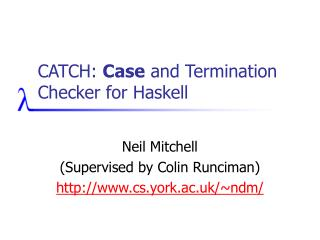DownloadDownload PresentationCATCH: Case and Termination Checker for Haskell

# CATCH: Case and Termination Checker for Haskell

Télécharger la présentation## CATCH: Case and Termination Checker for Haskell

- - - - - - - - - - - - - - - - - - - - - - - - - - - E N D - - - - - - - - - - - - - - - - - - - - - - - - - - -
##### Presentation Transcript

1. CATCH: Case and Termination Checker for Haskell Neil Mitchell (Supervised by Colin Runciman) http://www.cs.york.ac.uk/~ndm/

2. The Aim • Take a Haskell program • Analyse it • Prove statically that there are no “unsafe pattern matches” • No additional user work • Termination – not in 18 minutes!

3. Is this safe? risers [] = [] risers [x] = [[x]] risers (x:y:etc) = if x <= y then (x:s):ss else [x]:(s:ss) where (s:ss) = risers (y:etc)

4. Yes risers [] = [] risers [x] = [[x]]-– ((x:[]):[]) risers (x:y:etc) = if x <= y then (x:s):ss else [x]:(s:ss) where (s:ss) = risers (y:etc)

5. How does Catch work? • Transform to reduced Haskell • Apply transformations on reduced Haskell • Generate a condition for case safety • Propagate this condition • Figure out if the precondition is True

6. Pattern Matches let (a,b) = y in (b,a) if x then f else g f x | null x = [] | otherwise = tail x case x of [] -> True (a:b) -> a [x | Just x <- xs] f (x:xs) = x f x = ys where (y:ys) do (x:xs) <- f y return xs \(a,b) -> a ++ b f [x] = x

7. Reduced Haskell • Only simple case, functions, applications, constructors data [] = [] | (:) hd tl map f xs = case xs of [] -> [] (:) -> f xs.hd : map f xs.tl

8. Generating Reduced Haskell • Fully automatic • Uses Yhc’s Core language • Yhc is a fork of nhc98 • Specify –core or –corep to see it • Some additional transformations • Remove a few let’s • By the end, reduced Haskell

9. Transformations • About 8 are applied • Reachability • Eliminate dead code • Arity raising • Take out points free code • odd = not . even • Defunctionalisation [Reynolds 72] • Remove all higher order functions

10. The Checker itself • Operates on a simple first order language • Uses constraints of the form: • <expression, path, constructors> • From the expression, if I follow any valid path, I get to one of the constructors

11. Constraints, intro by example head (x:xs) = x <head@1, , {:}> fromJust (Just x) = x <fromJust@1, , {Just}> foldr1 f [x] = x foldr1 f (x:xs) = f x (foldr1 f xs) <foldr1@2, , {:}>

13. Dealing with recursion • Just keep expanding it • x ^ x.a ^ x.aa ^ x.aaa ^ x.aaaa • At a certain depth, give up • x.aaaa -> x.aaa* • Simplify after • x ^ x.a ^ x.aa ^ x.aaa ^ x.aaa* = x.a*

14. Going back to Risers <risers (y:etc), , {:}> <(y:etc), , {:}> True Risers is safe 

15. Other programs • Soda (Word search) • One minor tweak required • Was safe already • Adjoxo (XOX checker) • One fix requried • Was NOT safe before • Improves code readability

16. State of play • Have a working prototype • Full Haskell 98 • A number of Haskell 98 libraries • Works on 1-2 page programs • Still lots to do • A bit slow in some cases • Some programs don’t work yet

17. Conclusion • CATCH is a practical tool for detecting pattern match errors • Uses a constraint language to prove safety • http://www.cs.york.ac.uk/~ndm/ • A release is coming soon (2 months)

18. Transformation rules

19. Yhc vs GHC Core • GHC Core is: • More complex (letrec’s, lambda’s) • Lacks source position information • Piles and piles of type information • Slower to generate • Harder to change GHC • Less like the original code